# PSAT Math : How to multiply exponents

## Example Questions

1 3 Next →

### Example Question #493 : Algebra

Ifandare nonzero numbers such that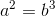, which of the following is equivalent to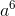?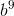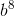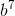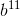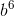Explanation:

For this problem, we need to make use of the property of exponents, which states that (xy)z = xyz.

We are given a2 but are asked to find a6.

Let's raise both sides of the equation to the third power, so that we will end up with a6 on the left side.

(a2)3 = (b3)3

Now, according to the property of exponents mentioned before, we can multiply the exponents.

a(2*3) = b(3*3)

a6 = b9

### Example Question #492 : Algebra

Ifandare positive integers and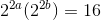, what is the value of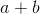?Explanation:

The question tells us that 22a ( 22b )= 16.

We can rewrite 16 as 24, giving us 22a ( 22b )= 24.

When terms with the same base are multipled, their exponents can be added:

2(2a +2b) = 24

Since the base is the same on both sides of the equation, we can equate the exponents:

2a +2b = 4

2(a + b) = 4

a + b = 2

### Example Question #21 : How To Multiply Exponents

Simplify: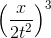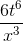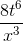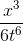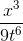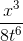Explanation:

Apply the the various properties of exponents: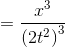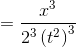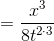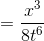### Example Question #51 : Exponents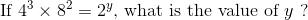Explanation: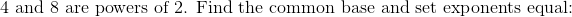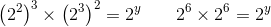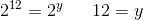### Example Question #61 : Exponents

Simplify: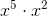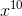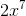This expression cannot be simplified any further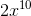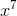Explanation:

When you are multiplying and the bases are the same, you add the exponents together. Because both bases areyou add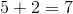as your new exponent. You then keep the same base,, to the 7th power.

### Example Question #61 : Exponents

Solve for: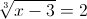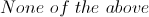Explanation: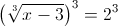Now the left side equals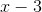and the right side equals 8.  Hence: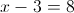Thereforemust be equal to 11.

1 3 Next →

### All PSAT Math Resources